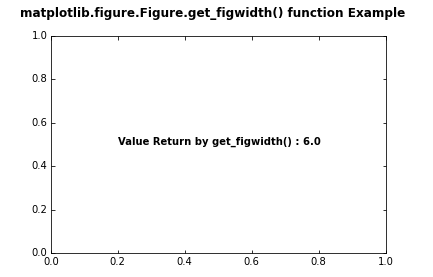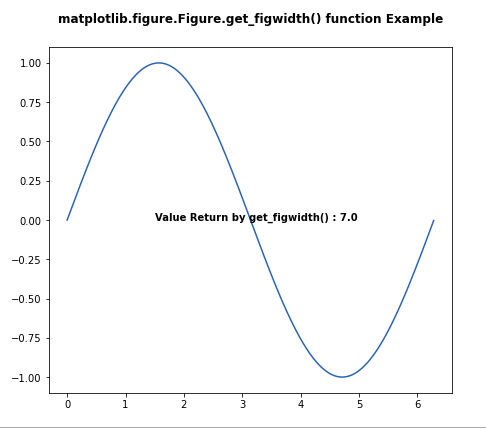Related Articles

# Matplotlib.figure.Figure.get_figwidth() in Python

• Last Updated : 30 Apr, 2020

Matplotlib is a library in Python and it is numerical – mathematical extension for NumPy library. The figure module provides the top-level Artist, the Figure, which contains all the plot elements. This module is used to control the default spacing of the subplots and top level container for all plot elements.

## matplotlib.figure.Figure.get_figwidth() method

The get_figwidth() method figure module of matplotlib library is used to get the figure width as a float.

Syntax: get_figwidth(self)

Parameters: This method does not accept any parameters.

Returns: This method return the figure width as a float.

Below examples illustrate the matplotlib.figure.Figure.get_figwidth() function in matplotlib.figure:

Example 1:

 `# Implementation of matplotlib function ``import` `matplotlib.pyplot as plt ``from` `matplotlib.figure ``import` `Figure``from` `mpl_toolkits.axisartist.axislines ``import` `Subplot ``import` `numpy as np `` ` ` ` `fig ``=` `plt.figure() ``     ` `ax ``=` `Subplot(fig, ``111``) ``fig.add_subplot(ax)  `` ` `w ``=` `fig.get_figwidth()``ax.text(``0.2``, ``0.5``, ``        ``"Value Return by get_figwidth() : "``        ``+` `str``(w),``        ``fontweight ``=``"bold"``)``   ` `fig.canvas.draw()``fig.suptitle('matplotlib.figure.Figure.get_figwidth() \``function Example', fontweight ``=``"bold"``) `` ` `plt.show()`

Output:Example 2:

 `# Implementation of matplotlib function ``import` `matplotlib.pyplot as plt ``from` `matplotlib.figure ``import` `Figure``import` `numpy as np `` ` ` ` `fig ``=` `plt.figure(edgecolor ``=` `"red"``, figsize ``=``(``7``, ``6``)) ``      ` `ax ``=` `fig.add_axes([``0.1``, ``0.1``, ``0.8``, ``0.8``])``    ` `xx ``=` `np.arange(``0``, ``2` `*` `np.pi, ``0.01``) ``ax.plot(xx, np.sin(xx)) ``  ` `w ``=` `fig.get_figwidth()``ax.text(``1.5``, ``0``,``        ``"Value Return by get_figwidth() : "``        ``+` `str``(w),``        ``fontweight ``=``"bold"``)``    ` `fig.canvas.draw()``fig.suptitle('matplotlib.figure.Figure.get_figwidth() \``function Example', fontweight ``=``"bold"``) `` ` `plt.show()`

Output:Attention geek! Strengthen your foundations with the Python Programming Foundation Course and learn the basics.

To begin with, your interview preparations Enhance your Data Structures concepts with the Python DS Course. And to begin with your Machine Learning Journey, join the Machine Learning – Basic Level Course

My Personal Notes arrow_drop_up